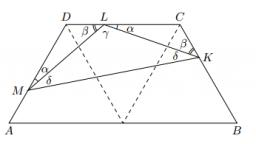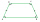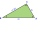# Internal angles

The ABCD is an isosceles trapezoid, which holds:
|AB| = 2 |BC| = 2 |CD| = 2 |DA|:

On its side BC is a K point such that |BK| = 2 |KC|, on its side CD is the point L such that |CL| = 2 |LD|, and on its side DA the point M is such that | DM | = 2 |MA|. Determine the internal angles of the KLM triangle.

∠KLM =  120 °
∠LMK =  30 °
∠MKL =  30 °Did you find an error or inaccuracy? Feel free to write us. Thank you!

Showing 1 comment:Math student
Help. First, look at the inner angles of the ABCD trapezoid.

Solution. It follows from the assumptions that the center line of the AB segment with the vertices C and D divides the ABCD trapezoid into three identical equilateral triangles. Therefore, the magnitude of internal angles in the trapezoid at A and B vertices is equal to 60 °
And at the C and D vertices 120 °. It follows from the specification that the triangles LCK and MDL are the same (according to the sentence above). Therefore, both the KL and LM lines and the designated pairs of angles are the same; The magnitudes of these angles are denoted α and β. The triangle KLM is isosceles and the angles at the base are the same; Their size is denoted by δ and the size of the angle KLM is denoted by γ.

From the sum of the inner angles in the KCL triangle we derive
α + β = 180° − 120° = 60°

The sum of the three marked angles with the vertex L is a straight angle, therefore
γ = 180° − (α + β) = 120°
Finally, we deduce the sum of inner angles in the triangle KLM
δ = (180° − 120°)/2 = 30°

The internal angles of the triangle KLM are 30° and 120°Tips to related online calculators

#### You need to know the following knowledge to solve this word math problem:

We encourage you to watch this tutorial video on this math problem:

## Related math problems and questions:

• MO Z7–I–6 2021In the triangle ABC, point D lies on the AC side and point E on the BC side. The sizes of the angles ABD, BAE, CAE and CBD are 30°, 60°, 20° and 30°, respectively. Find the size of the AED angle.
• Inner anglesThe magnitude of the internal angle at the main vertex C of the isosceles triangle ABC is 72°. The line p, parallel to the base of this triangle, divides the triangle into a trapezoid and a smaller triangle. How big are the inner angles of the trapezoid?
• Isosceles - isoscelesIt is given a triangle ABC with sides /AB/ = 3 cm /BC/ = 10 cm, and the angle ABC = 120°. Draw all points X such that true that BCX triangle is an isosceles and triangle ABX is isosceles with the base AB.
• Isosceles trapezoid v3In an isosceles trapezoid ABCD is the size of the angle β = 81° Determine size of angles α, γ and δ.
• MO Z8–I–6 2018In the KLMN trapeze, KL has a 40 cm base and an MN of 16 cm. Point P lies on the KL line so that the NP segment divides the trapezoid into two parts with the same area. Find the length of the KP line.
• Equilateral triangle ABCIn the equilateral triangle ABC, K is the center of the AB side, the L point lies on one-third of the BC side near the point C, and the point M lies in the one-third of the side of the AC side closer to the point A. Find what part of the ABC triangle cont
• Two forcesTwo forces with magnitudes of 25 and 30 pounds act on an object at 10° and 100° angles. Find the direction and magnitude of the resultant force. Round to two decimal places in all intermediate steps and your final answer.
• Acute triangleIn the acute triangle KLM, V is the intersection of its heights and X is the heel of height to the side KL. The axis of the angle XVL is parallel to the side LM and the angle MKL is 70°. What size are the KLM and KML angles?
• AnglesThe outer angle of the triangle ABC at the vertex A is 114°12'. The outer angle at the vertex B is 139°18'. What size is the internal angle at the vertex C?
• Outer anglesThe outer angle of the triangle ABC at the A vertex is 71°40 ' outer angle at the vertex B is 136°50'. What size has the inner triangle angle at the vertex C?
• TrianglesFind out whether given sizes of the angles can be interior angles of a triangle: a) 23°10',84°30',72°20' b) 90°,41°33',48°37' c) 14°51',90°,75°49' d) 58°58',59°59',60°3'
• Trapezoid thirdsThe ABCD trapezoid with the parallel sides of the AB and the CD and the E point of the AB side. The segment DE divides the trapezoid into two parts with the same area. Find the length of the AE line segment.
• MO - trianglesOn the AB and AC sides of the triangle ABC lies successive points E and F, on segment EF lie point D. The EF and BC lines are parallel and is true this ratio FD:DE = AE:EB = 2:1. The area of ABC triangle is 27 hectares and line segments EF, AD, and DB seg
• Diamond and anglesFind the area of a diamond with a side of 5 cm if you know that the internal angles in the diamond are 60° and 120°.
• Inner anglesThe inner angles of the triangle are 30°, 45° and 105° and its longest side is 10 cm. Calculate the length of the shortest side, write the result in cm up to two decimal places.
• RWYCalculate the opposite direction of the runway 13. Runways are named by a number between 01 and 36, which is generally one tenth of the azimuth of the runway's heading in degrees: a runway numbered 09 points east (90°), runway 18 is south (180°), runway 2
• Triangle ABCIn a triangle ABC with the side BC of length 2 cm The middle point of AB. Points L and M split the AC side into three equal lines. KLM is an isosceles triangle with a right angle at the point K. Determine the lengths of the sides AB, AC triangle ABC.